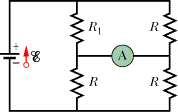# Getting currents in ammeter, with some resistances.

dhkdeoen

## Homework StatementIn the figure R1 = 1.29R, the ammeter resistance is zero, and the battery is ideal. What multiple of ε/R gives the current in the ammeter?

## Homework Equations

V=IR, 1/R=1/R_1+1/R_2..(when resistances are parallel)
etc

## The Attempt at a Solution

since ammeter doesn't have resistance, two top resistances and two bottom resistances are basically parallel. First, I set the currents coming out from the battery is i, and I was getting R_eq

R_top: R_1+R=(119/229)R
R_bottom: R/2
R_eq=R_top+R_bottom=(467/458)R

set the voltage as ε

ε=(467/458)*iR
ε=V_top+V_bottom=V_top+iR
(since two bottom resistances are same, so V_bottom=iR)

(467/458)*iR=V_top+iR
V_top=(467/458)*iR-iR=(9/458)*iR

and

i_topleft=V_top/1.29R=(9/458)iR*1/(1.29R)=(0.015233...)*i
i_A=current through ammeter
i_A+i_topleft=i
i_A=i-(0.015233..)i=(0.9847..)i

so I got 0.9847.. for the answer, which is wrong. where did I go wrong? I spent like 3 hours on this problem, and still can't get it.

## Answers and Replies

Homework Helper
The equivalent top resistance is not 119/229 R.

It is also wrong that Vbottom=iR.

It is better to do the derivation symbolically, and substitute the numerical data at the end.

ehild

dhkdeoen
I couldn't managed to pull out the answer, so I did what you said.
R_t=(R*R1)/(R+R1)
R_b=R/2

R_eq=(R*R1)/(R+R1)+R/2

ε=R_eq*i

ε=V_t+V_b=V_t+0.5iR
V_t=R_eq*i-0.5iR
i_topleft=V_t/(1.29R)

so
i_A+i_topleft=i
i_A=i-i_topleft

i_A=i-(V_t/(1.29R))=i-((R_eq*i-0.5iR)/(1.29R))=i-(((R*R1)/(R+R1)+R/2*i-0.5iR)/(1.29R))
R1=R*1.29

i_A=i-((1/2.29+1/2)i-0.5i)/1.29
since I won't need i in the answer
A=1-((1/2.29+1/2)-0.5)/1.29)=0.66148
http://www.wolframalpha.com/input/?i=1-((1/2.29+1/2)-0.5)/1.29

which is still wrong. I'll appreciate your correction on my attempt.

Homework Helper
I couldn't managed to pull out the answer, so I did what you said.
R_t=(R*R1)/(R+R1)
R_b=R/2

R_eq=(R*R1)/(R+R1)+R/2

ε=R_eq*i

ε=V_t+V_b=V_t+0.5iR
V_t=R_eq*i-0.5iR
i_topleft=V_t/(1.29R)

so
i_A+i_topleft=i

The equation in red is wrong if iAmeans the current through the ammeter.

ehild

dhkdeoen
I just realized I've mixed up my assumptions.

i_A+i_topleft=0.5i

is this right?
and, yes i_A is the current through the ammeter.

Homework Helper
I just realized I've mixed up my assumptions.

i_A+i_topleft=0.5i

is this right?
and, yes i_A is the current through the ammeter.

Yes, it is correct.

ehild

dhkdeoen
then it should be

i_A=0.5i-(((R*R1)/(R+R1)+R/2)*i-0.5iR)/(1.29R))

substitute R1=1.29R

i_A=0.5i-(1.06332iR-0.5iR)/(1.29R)
i_A=0.5i-(0.56332/1.29)i
i_A=0.5i-0.43668217i
i_A=0.06331877i

I=V/R
i=ε/R

so this should be the answer, but still..

Last edited:
Homework Helper
You made it a bit complicated. ((R*R1)/(R+R1)+R/2)*i=ε.

It is simpler with the topright current.
iA=i/2-itopright.
itopright=Vt/r
Vt=ε-ir/2.
-->

iA=i-ε/r.

i=ε/Req, and Req=r(3r1+r)/(2(r+r1)). ..

ehild

Last edited:
•1 person
Mentor
Question: Have you studied Thevenin equivalents yet?

dhkdeoen
You made it a bit complicated. ((R*R1)/(R+R1)+R/2)*i=ε.

It is simpler with the topright current.
iA=i/2-itopright.
itopright=Vt/r
Vt=ε-ir/2.
-->

iA=i-ε/r.

i=ε/Req, and Req=r(3r1+r)/(2(r+r1)). ..

ehild

it is! Maybe I was too hanging out with R1. Now I got the right answer.
Even though I got the right answer, I really should trying to get answer with R1, though. So much appreciated for your devotion!

dhkdeoen
Question: Have you studied Thevenin equivalents yet?

I don't think I've heard of that. My class just started with Kirchhoff's two rules.

Homework Helper
then it should be

i_A=0.5i-(((R*R1)/(R+R1)+R/2)*i-0.5iR)/(1.29R))

substitute R1=1.29R

i_A=0.5i-(1.06332iR-0.5iR)/(1.29R)
i_A=0.5i-(0.56332/1.29)i
i_A=0.5i-0.43668217i
i_A=0.06331877i

I=V/R
i=ε/R

so this should be the answer, but still..

You need the answer in term of ε/R, but i=ε/Req

By the way, the derivation is much simpler if you simplify whenever possible.

Vt= i(R1R)/(R1+R), Itopleft=Vt/R1=iR/(R1+R),
iA=i/2-iR/(R1+R)= i(R-R1)/(2(R+R1))...

ehild

Mentor
I don't think I've heard of that. My class just started with Kirchhoff's two rules.
Okay. I was just wondering what level of theory you'd covered. While problems like this one can be solved with basic Kirchcoff's laws, they become easier to tackle when you've covered some additional techniques. I thought perhaps it might have been an exercise intended to evoke such an approach. Never mind, carry on